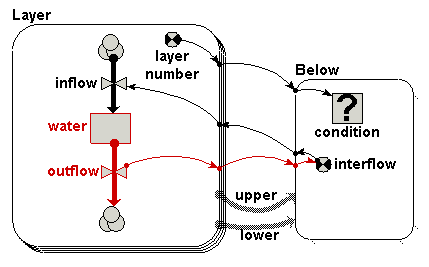# Flow between layers - using association submodel

This template is for modelling the flow of some substance between multiple layers.  A typical application would be for modelling the flow of, for example, water or heat between soil layers.

The basic idea is to have a multiple-instance submodel for the layers, with each instance representing a single layer.   Each layer has a single compartment (stock) representing the amount of substance in that layer.   At least two flows are associated with teh compartment, an inflow representing the rate at which the substance flows into the layer from the layer above, and an outflow representing the rate at which the substance flows into the layer below.

I say "at least two flows...", because the modeller might choose to add additonal flows, e.g. for rainfall into the first layer and drainage flow out of the last layer.  Alternatively, the inflow into the top layer can be considered to be rainfall, and the outflow from the bottom layer can be considered to be drainage out of the system.

We need to link the outflows and inflows of neighbouring layers together, so that the inflow of layer i+1 is set equal to the outflow of layer i (counting the top layer as number 1).   There are two ways of doing this.  One method is to use an array variable outside the submodel: this should be illustrated in another template.  In this template, however, we use an association submodel to link neighbouring layers together.  This has considerable benefits when there are many layers, since Simile needs to handle only as many values as there are interflows between layers.## Equations

Below/condition = layer_number_lower==layer_number_upper+1

Below/interflow = outflow_lower

Layer/inflow = sum({interflow_upper})

thumbnail: